# 桌球瞄准系统2.0

2018-09-20 ` 约 1655 字 ` ` 预计阅读 4 分钟 `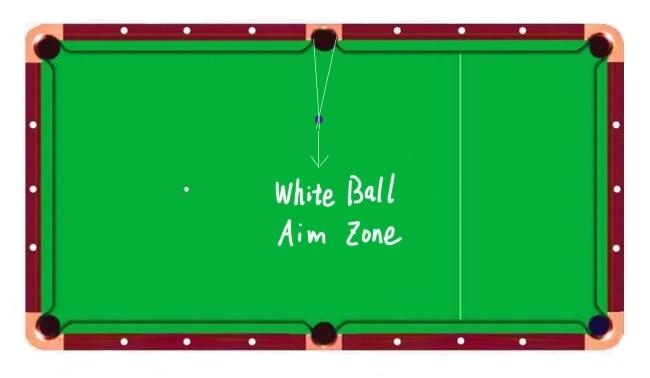由上图看，只要白球打倒了画出来的那个小三角区域，目标球就能进袋。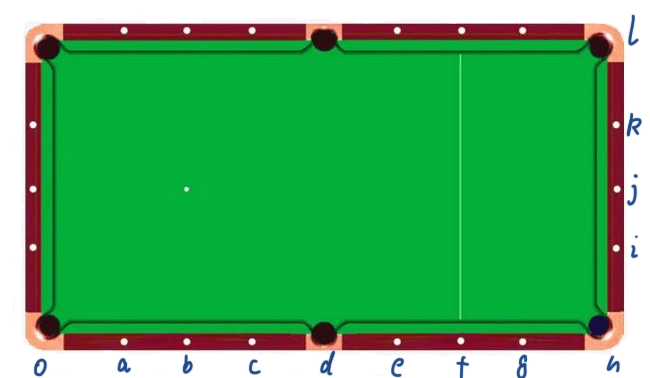∠iOh=arctan(1/8)=7度

∠jOh=arctan(2/8)=14度

∠kOh=arctan(3/8)=21度

∠lOh=arctan(4/8)=27度

∠glh=arctan(0.25)=14度

∠flh=arctan(0.5)=27度

∠elh=arctan(0.75)=37度

∠dlh=arctan(1)=45度

∠clh=arctan(1.25)=51度

∠blh=arctan(1.5)=56度

∠alh=arctan(1.75)=60度

∠olh=arctan(2)=63度

OK，这样所有的角度应该都能背熟了。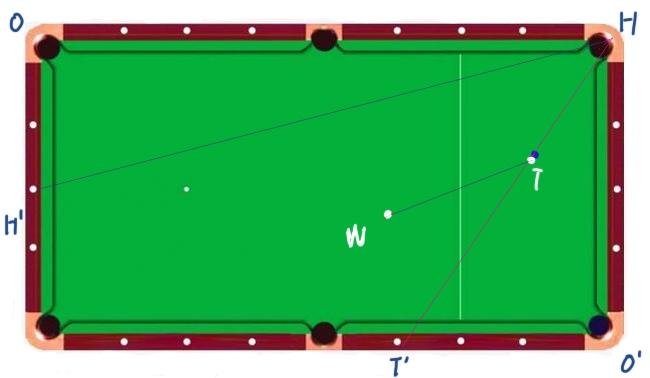∠O’HT=36度

H’点在短边情人节位置，则
∠OHH‘=14度

∠WTT‘=90-36-14=40度

1.0版本算出来的38度略有差距。

1.0版已经计算了各个点对T点及H点的锐角。放在这里：
a点为72度。

b点是45度。

c点是63-45=18度。

d点为0度。不需要考虑

e点为45-arctan(2/3)=45-33=12度

f点为45-arctan(2/4）=45-27=18度。跟c点一样，只是从另一方向瞄准。

g点为45-arctan(2/5)=45-22=23度。

h点为45-arctan(2/6)=45-18=27度

i点为45-arctan(1/6)=45-9=36度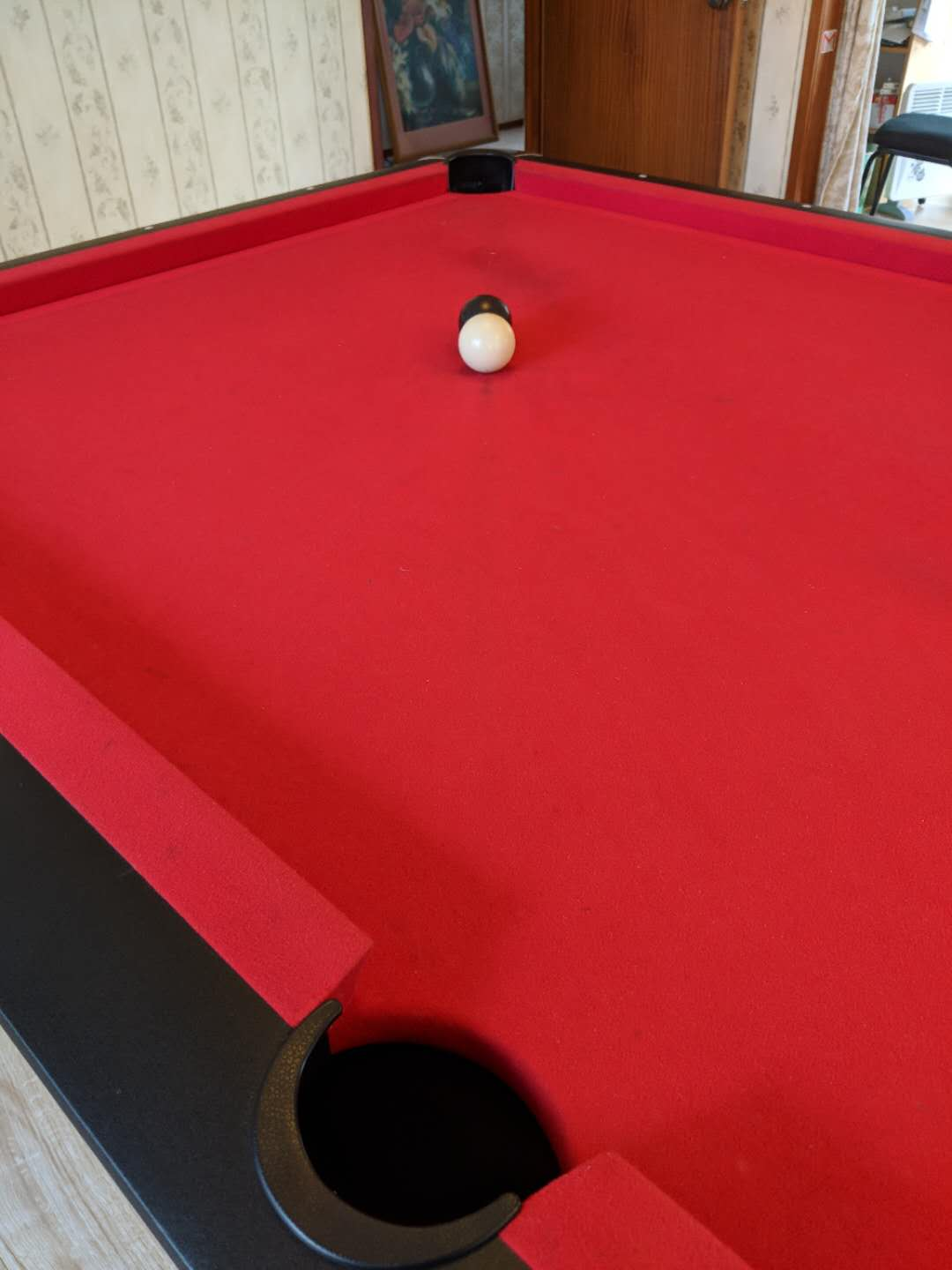a点72度，瞄的位置基本要偏半个球，难度很大。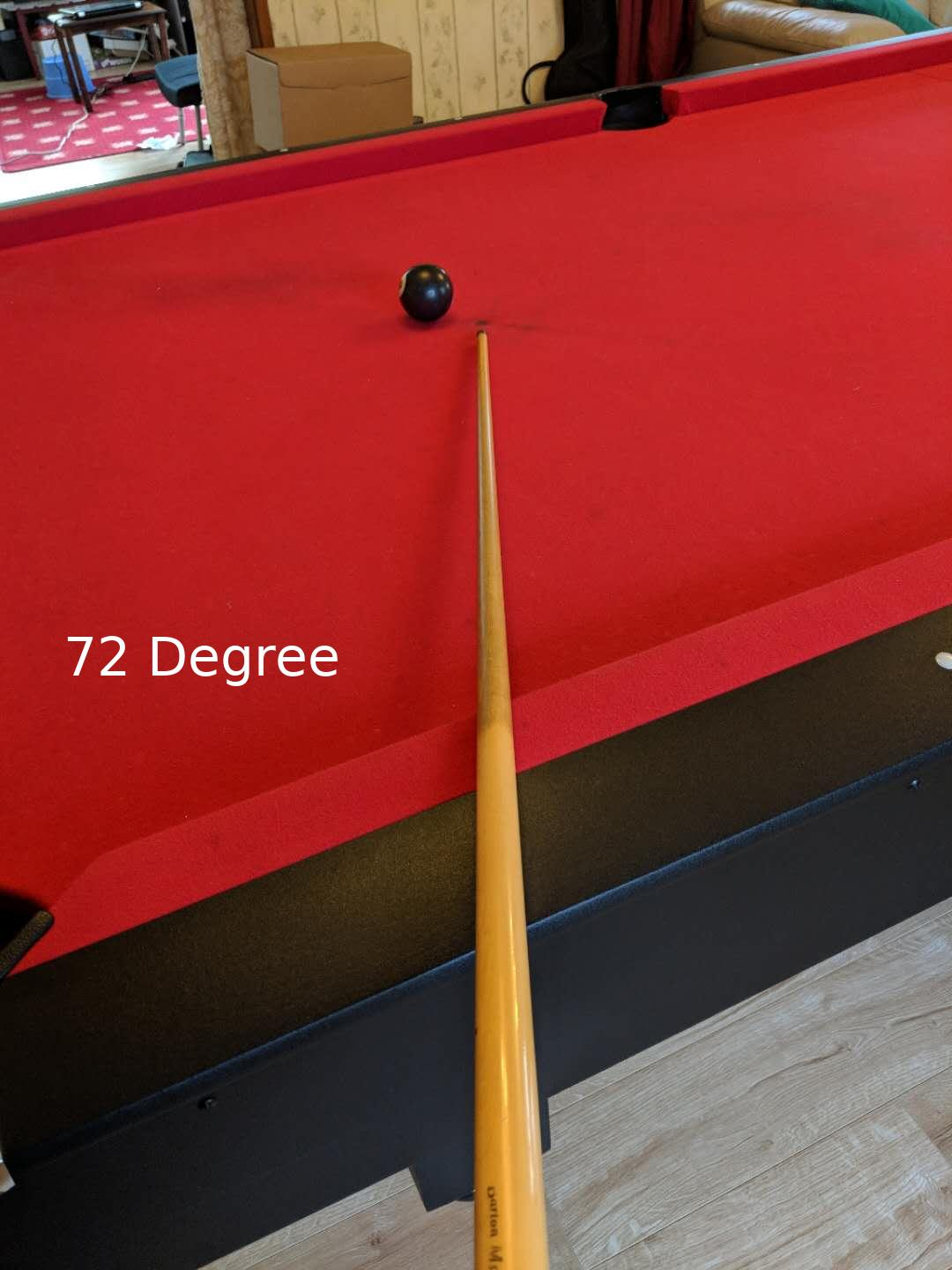b点45度，瞄的位置是黑球外侧两杆宽左右。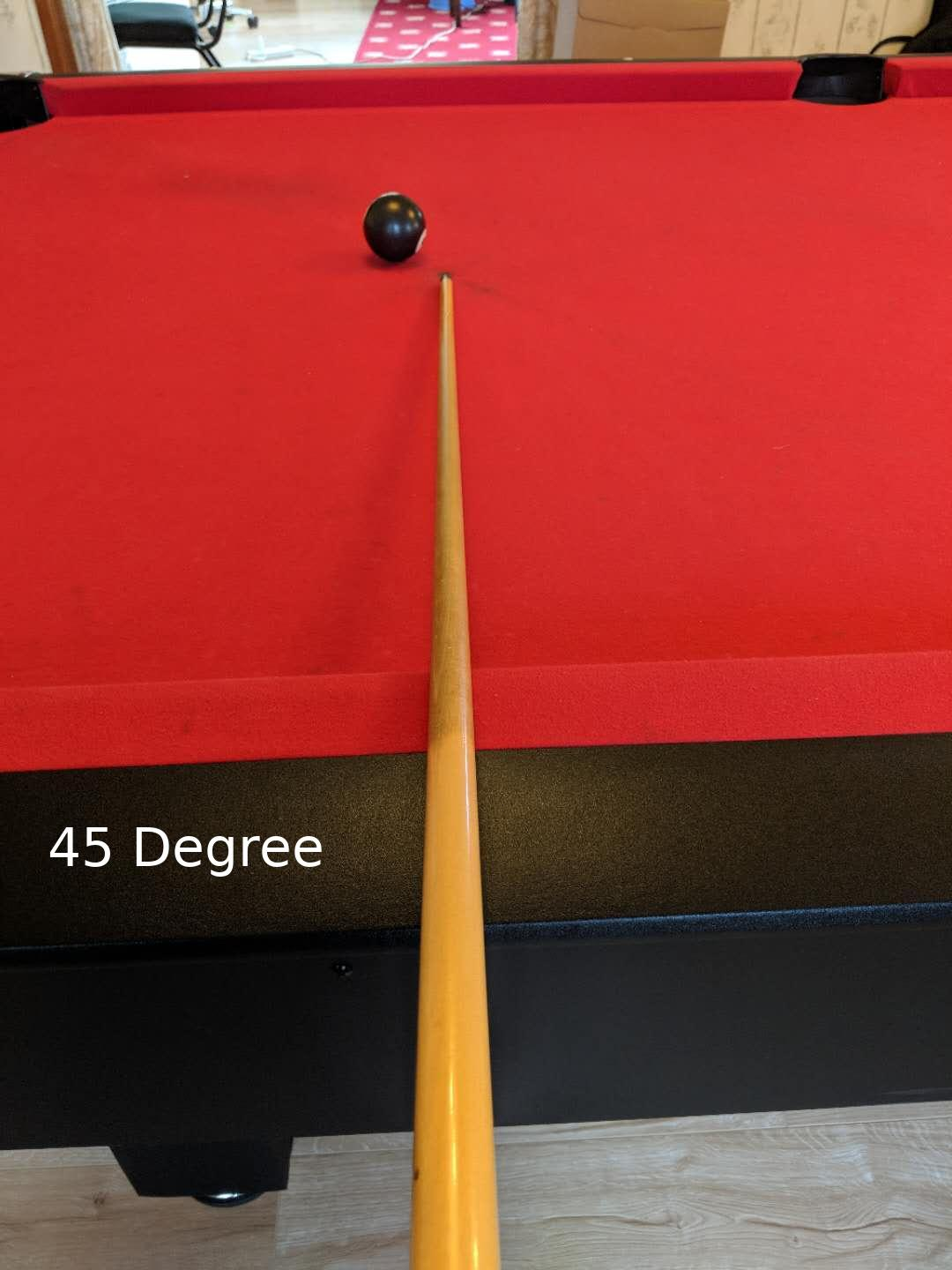c点18度，瞄的位置是将杆的右侧与黑球外侧基本对齐即可。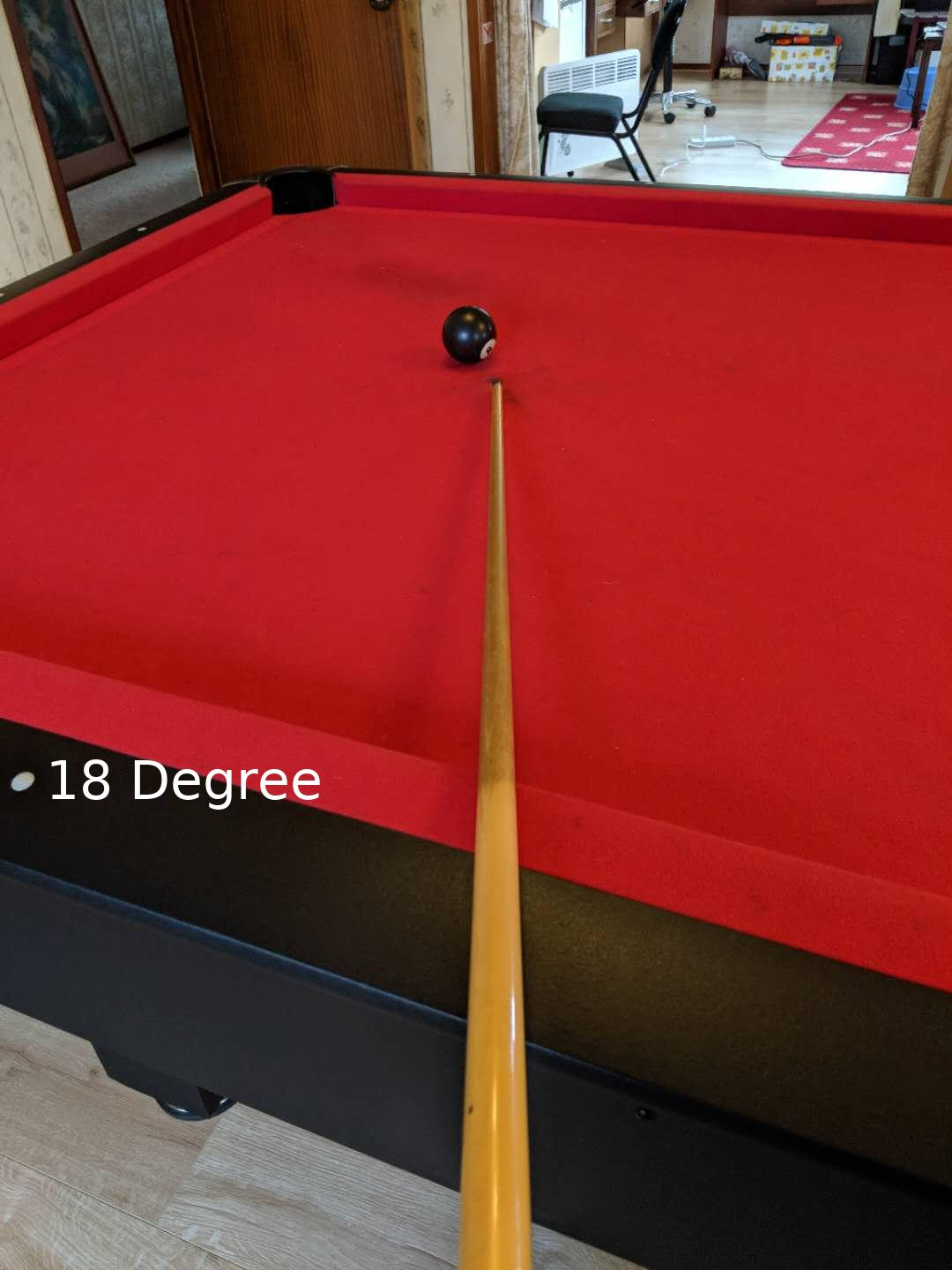e点12度，杆正中心在黑球2/5处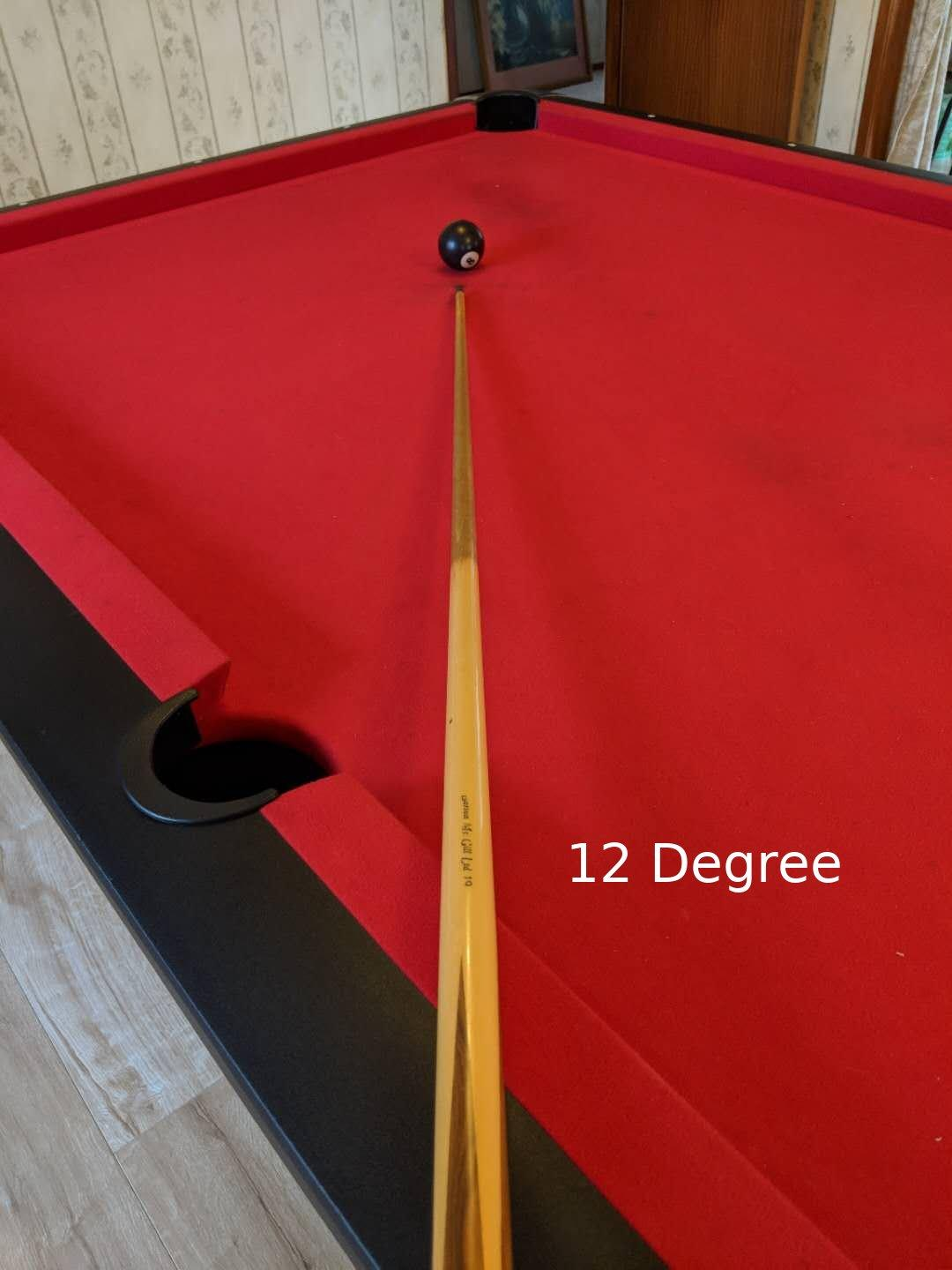g点23度，杆中心在黑球1/5左右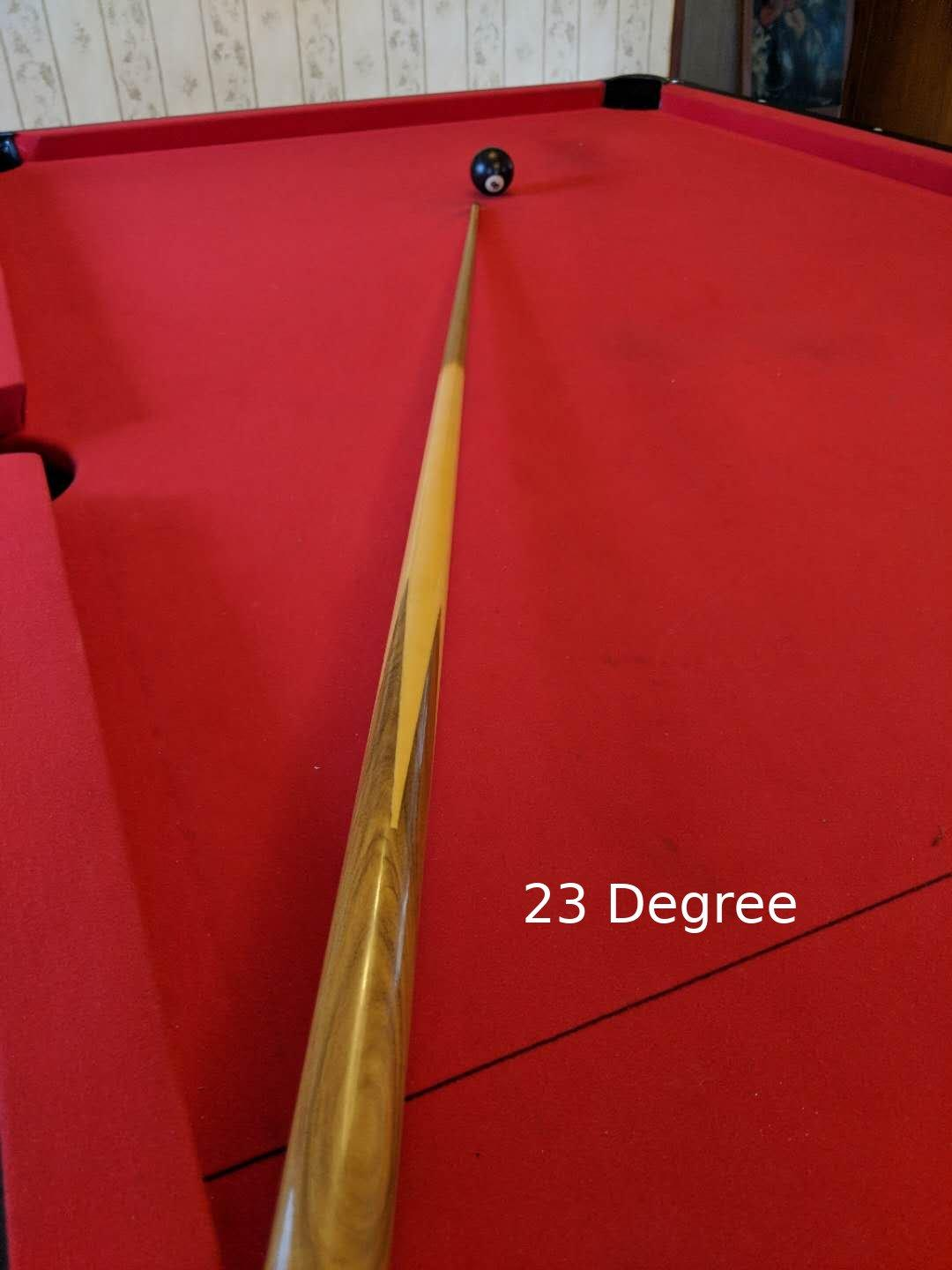h点27度，基本就是瞄准黑球外侧。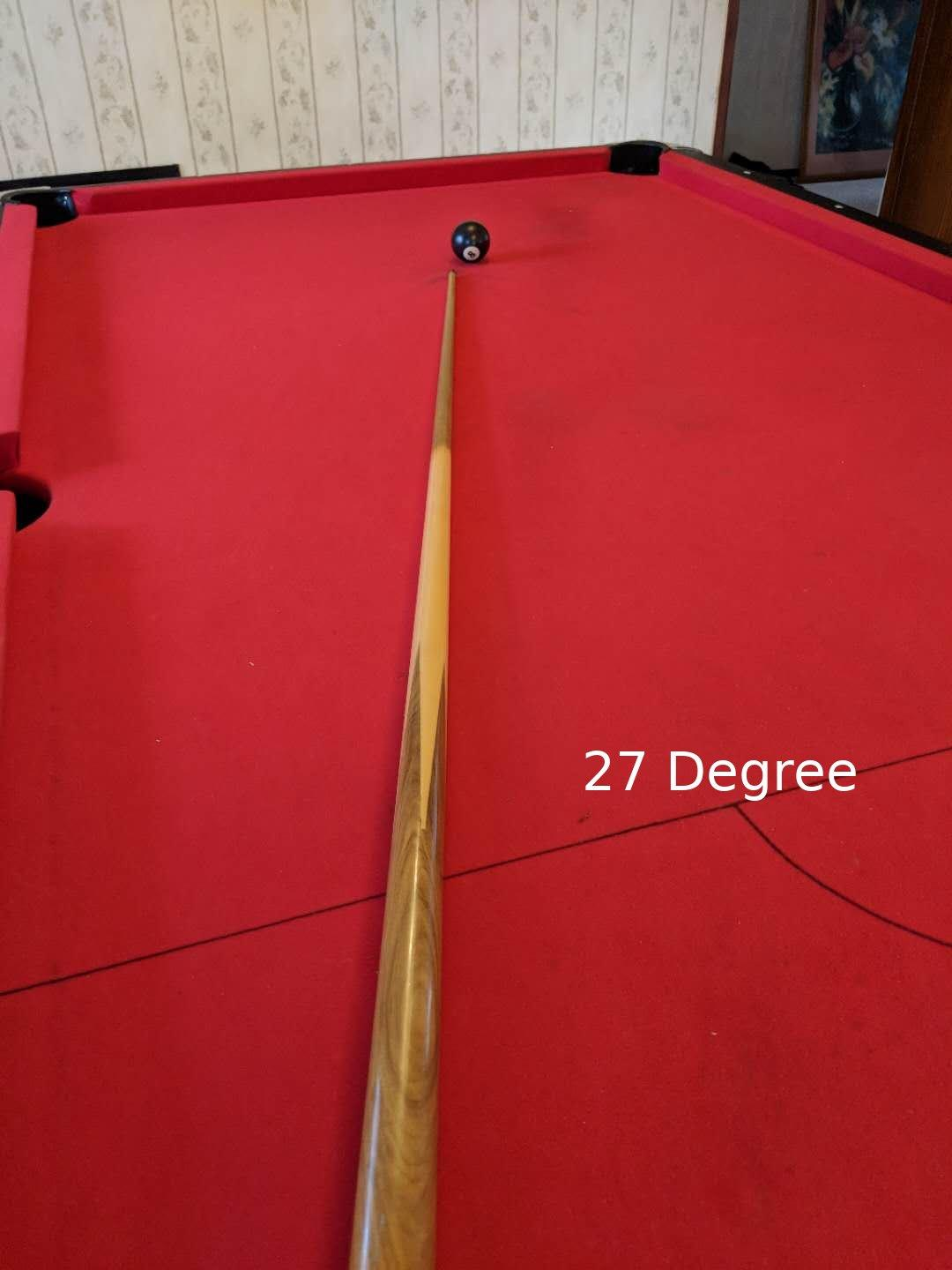i点36度，基本就是杆外侧与黑球外侧基本对齐。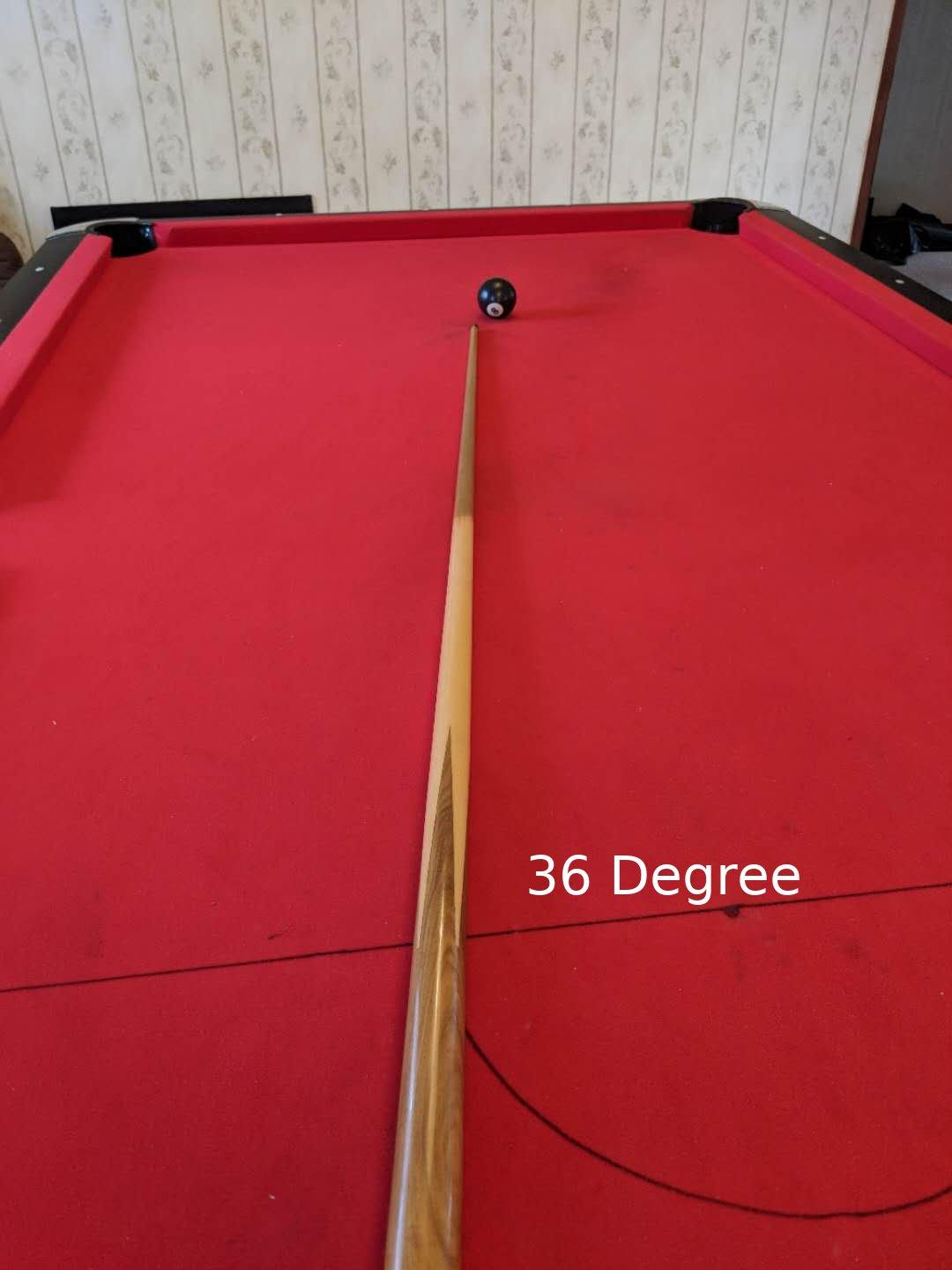60度，我们记得长边倒数第二个是乾隆60，故此可以摆出一个60度角的球。貌似跟45度角差不太多。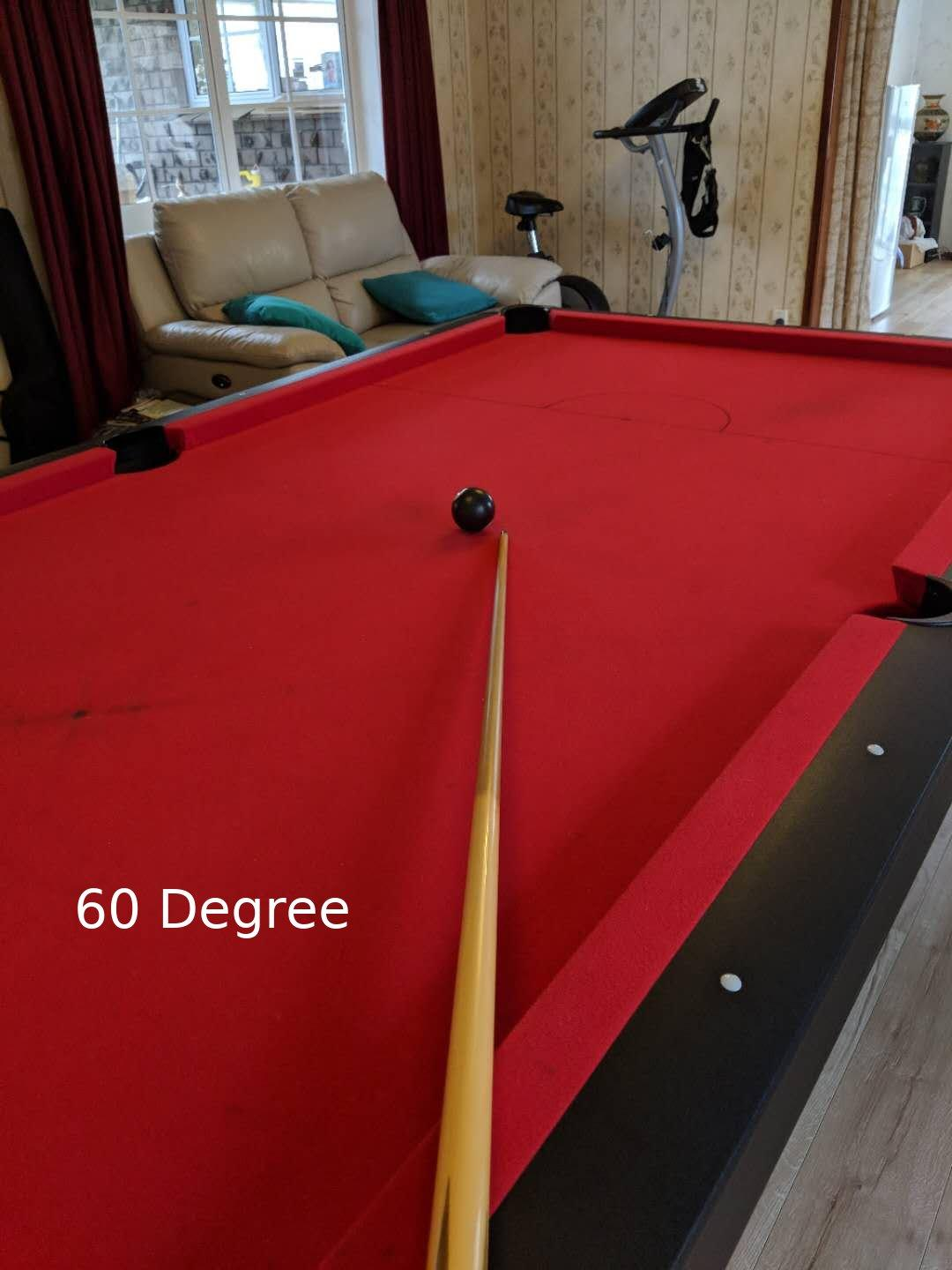Tags: 台球